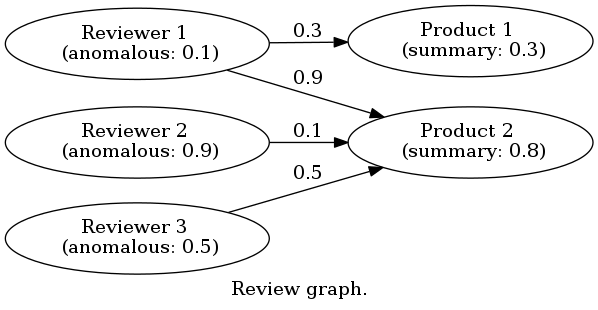# Review Graph Mining¶A framework of review data mining based on a graph model.

It helps both data analysts who want to analyze their review data, and research scientists who want to make other mining algorithms.

For data analysts, you can easily compare several algorithms:

All packages are also available on PyPI and you can install them by pip install.

To use one algorithm, you need to create a review graph object provided by each package, create reviewers and products, add then run the algorithm. For example, the following code shows a way to use MRA algorithm with the above review graph:

# Construct a Review Graph instance.
import ria
graph = ria.mra_graph()

# Create reviewers and products.
reviewers = [graph.new_reviewer("reviewer-{0}".format(i)) for i in range(1,4)]
products = [graph.new_product("product-{0}".format(i)) for i in range(1,3)]

# Start the algorithm.
for i in range(10000):
# Run one iteration.
diff = graph.update()
print("Iteration %d ends. (diff=%s)", i + 1, diff)
# If the update becomes negligible, the algorithm ends.
if diff < 10**-5:
break

# Print results.
for r in graph.reviewers:
print(r.name, r.anomalous_score)
for p in graph.products:
print(p.name, p.summary)


If you want to analyze a dataset given by this project or your review data are formatted in the JSON format, you can run algorithms via analyze command provided by Scripts for Analyzing Review Graphs package.

For example, the following command analyzes the synthetic dataset by the Fraud Eagle algorithm with parameter $$\epsilon = 0.25$$:

$analyze synthetic feagle --method-param epsilon=0.25  To run the above command, you may need to install rgmining-script, rgmining-synthetic-dataset, and rgmining-fraud-eagle packages. Or the following command analyze your review data file review.json by the FRAUDAR algorithm with parameter blocks = 10: $ analyze file --dataset-param file=review.json fraudar --method-param blocks=10


To run the above command, you may need to install rgmining-script, and rgmining-fraudar packages.

For research scientists, you can evaluate your algorithms comparing with other algorithms. This project also provides several dataset loaders:

To use these loaders, you need to make a review graph object which implements the Graph interface.

For example, the following code shows a way to load the Six Categories of Amazon Product Reviews Dataset to a graph object graph:

import amazon
# graph must implement the graph interface.